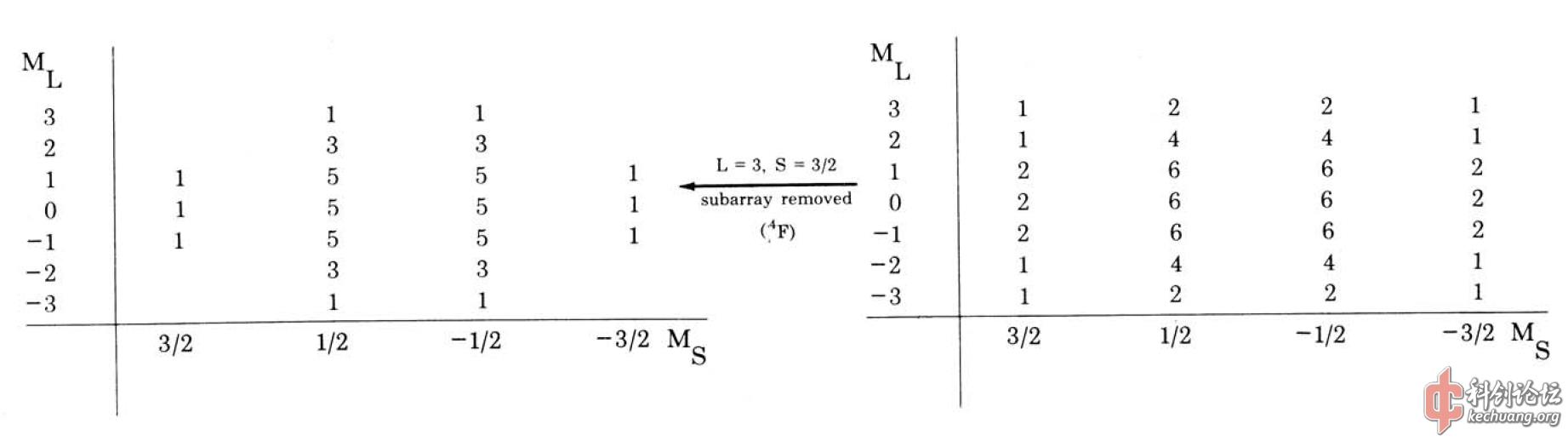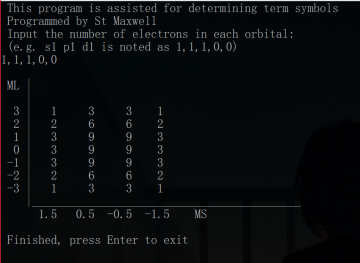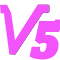求解电子光谱项的程序

本文原地址：东方红茶馆-求解电子光谱项的程序
与原文可能略有不同。

该程序可以处理几乎所有的电子组态（包含的轨道有$$s, p, d, f, g$$），如 $$({\rm s})^1({\rm p})^2({\rm d})^2$$这样的组态。但目前不能求解如 $$(2{\rm p})^2(3{\rm p})^2$$这一类组态的光谱项。

求原子光谱项有很多种方法，这里只介绍一种思路简单粗暴的方法。某种程度上可称为是逐一枚举的方法。（等你看完说不定会觉得这个方法很low23333）

其实该方法也可看作是结构化学教科书上讲解两个同科电子组态（$$(n\text{p})^2$$）的光谱项的方法的推广。

## 表格法求光谱项的原理

为什么某一组态的能级会发生分裂呢？这是因为该组态是简并的（没有某些扰动的理想条件下），而当考虑了扰动后，原本简并的微观能级不再简并而发生分裂。

我们知道，对于多电子原子中的任意一个电子，其状态可由其单电子原子轨道（波函数）近似描述，其中单电子波函数含有四个量子数。也就是说只要确定了这四个量子数，便能够确定其状态。而组态只能确定电子的主量子数 $$n$$和角量子数 $$l$$，并没有进一步描述电子的磁量子数 $$m$$和自旋磁量子数 $$m_s$$。

组态的能级分裂就是因为电子的磁量子数和自旋磁量子数的不同，虽然对于多电子原子来说使用总角量子数 $$L$$、总自旋量子数 $$S$$和总量子数 $$J$$的概念会更合适。

所以该方法的第一步是确定某一组态的所有微观能态。

以 $$\text{d}^3$$组态为例。我们首先要考虑其所有的电子排布方式。由于Pauli原理的限制，电子的状态没有完全相同的。d轨道有10个对应的自旋轨道，所以总的微观状态数为 $$\left( \begin{matrix} 10 \\ 3 \end{matrix} \right)=120$$。

以图所示的状态为例，三个电子的磁量子数分别为2、2、1，所以 $$M_L=5$$。而总自旋磁量子数 $$M_S=1/2$$。也就是我们要计算一共120种微观能态的 $$M_L$$和$$M_S$$。如果手算的话，显然这是一个很麻烦的事情。

当我们将120种组合的 $$M_L$$和 $$M_S$$都算出来后，就可绘制出 $$M_L$$-$$M_S$$表格。

以总自旋磁量子数为例，三个电子共有四种情况。电子的自旋全部相同以及其中一个电子与另外两个的自旋不同。所以 $$M_S=3/2, 1/2, -1/2, -3/2$$。

相应地，$$M_L=5, 4, 3,\ldots,-4, -5$$。我们将各个组合中具有相同 $$M_L$$、$$M_S$$分别计数，便可得到该表。然后只需要一步步从表中提取出光谱项即可。从最大的 $$M_L=5$$开始看。$$M_L$$为 $$L$$的最大值，$$L$$的取值范围为 $$0, 1,\ldots,\pm L$$。而且对应的 $$S(M_S)=1/2, -1/2$$。由此我们可推断出该组态有一 $$^2\text{H}$$的光谱项。

然后我们将表格中二三列的数字均减去1，将该光谱项移除，得到第二步的表。

下图右边的是第二次约去光谱项后得到的表，此时 $$L=3\ldots-3$$，而 $$S=3/2, 1/2, -1/2, -3/2$$。所以可提取出光谱项 $$^4\text{F}$$。最后得到的 $$\text{d}^3$$组态的所有的光谱项为：$$^2\text{H},\ ^2\text{G},\ ^4\text{F},\ ^2\text{F},\ ^2\text{D}(2),\ ^4\text{P},\ ^2\text{P}$$

以上方法适用于任何同科电子组态光谱项的求算。而对于存在不同壳层的组态（例如 $$\text{p}^2\text{d}^2$$），只需要先分别求得p轨道的电子以及d轨道的电子的组合，再将不同角量子数的组合再一次组合即可。以 $$\text{p}^2\text{d}^2$$组态为例，此时总的微观状态数为 $$\left( \begin{matrix} 6 \\ 2 \end{matrix} \right)\times\left( \begin{matrix} 10 \\ 2 \end{matrix} \right)=15\times 45=675$$。这一方法说不上很高效，但思路很简单。之后生成表格的步骤则是完全一样的。

## 代码的思路

我是用Fortran写的这个程序，源代码可在GitHub下载到。

下面主要介绍代码的思路。

首先以$$d$$轨道的同科电子组态为例。

d轨道共有10个自旋轨道，所以声明了两个含有10个元素的数组分别储存各自旋轨道的磁量子数和自旋磁量子数。

电子数是由用户输入的，然后依次列举所有的自旋轨道的组合并计算 $$M_L$$和 $$M_S$$并分别储存在两个数组中。这里需要注意，由于电子数并非事先写在程序中，所以列举组合的部分无法直接使用嵌套的循环来做到（订正：实际上是可以的）。这里使用的是一个利用递归的子程序来实现的。

然后统计 $$M_L$$和 $$M_S$$的取值各有多少种，并生成两个数组分别储存这些值（按由大到小的顺序依次储存）。

例如：120种组合的 $$M_L$$依次为 $$(5, 3, 2, 3, -1, 5, 4, -2,...)$$，而之后的那个数组为 $$(5, 4, 3, 2,...,-4, -5)$$ 这11个值。

我们将前一个数组中的120个值与后一个数组进行比较，记录各个值出现的次数，便能够生成之前那个表中的数据。

对于具有不同角量子数的轨道的组态来说，则是分别将各个轨道上的组合再一次组合一次。 运行示例：关于直接输出光谱项的功能的实现

由于直接对输出的表格进行操作很麻烦，而且也不必要（因为对整个表格操作需要的循环实现困难，而且表无论是行还是列都是对称的）。所以为了实现该功能，我首先取原有的表格的左上部分单独存为一个如图所示的二维数组。之后检查第一行各列的数字 $$n$$，若为零，则跳过；若非零，则根据其对应的总角量子数 $$L$$和总自旋角量子数 $$S$$得到其光谱项（若不为1，输出的光谱项后会有类似<n>的标记，表明该光谱项的数目为 $$n$$），之后的各行与各列都减去 $$n$$。一行结束后，进入下一行，重复以上的步骤。（没看明白的话，可以按照第一节的例子跟着手算一下）

最终得到的结果是对于多个壳层的情况（例如 $$p^2d^2$$），则是分别计算各壳层的所有组合，再按壳层进一步组合获得所有可能的情况。如果理解了前面的步骤，这一步并不难理解。之后确定光谱项的方法是完全一样的。

多个相同角动量相同的壳层也是可以计算的，如## 参考文献

1. Journal of Chemical Education, 52(2), 1975:87-89
2. 大学化学, 29(2), 2014:44-46

[修改于 2 年前 - 2019-08-29 21:52:32]

来自：数理化 / 化学

想参与大家的讨论？现在就 登录 或者 注册

所属专业
所属分类
上级专业
同级专业St_Maxwell进士 学者 机友 笔友
文章
29
回复
823
学术分
6
2012/03/11注册，1 个月前活动

Bachelor's Degree, Chemistry, Sichuan University

Ph.D Student, Theoretical and Computational Chemistry, Xiamen University

Site:东方红茶馆

%7B%22isDisplay%22%3Atrue%7D
视频暂不能访问，请登录试试
仅供内部学术交流或培训使用，请先保存到本地。本内容不代表科创观点，未经原作者同意，请勿转载。
音频暂不能访问，请登录试试
文件下载
加载中...
{{errorInfo}}
文件名称：{{resource.defaultFile.name}}
下载次数：{{resource.hits}}
所需积分：{{costScores}}，{{holdScores}}下载当前附件免费{{description}}
积分不足，去充值
文件已丢失

##### 当前账号的附件下载数量限制如下：
时段 个数
{{f.startingTime}}点 - {{f.endTime}}点 {{f.fileCount}}
插入资源
全部
图片
视频
音频
附件
全部
未使用
已使用
正在上传
空空如也~
上传中..{{f.progress}}%
处理中..
上传失败，点击重试
等待中...
{{f.name}}
空空如也~
(视频){{r.oname}}
{{selectedResourcesId.indexOf(r.rid) + 1}}
处理中..
处理失败
插入表情
我的表情
共享表情
Emoji
上传
##### 注意事项
最大尺寸100px，超过会被压缩。为保证效果，建议上传前自行处理。
建议上传自己DIY的表情，严禁上传侵权内容。
点击重试等待上传{{s.progress}}%处理中...已上传
空空如也~
草稿箱
加载中...
此处只插入正文，如果要使用草稿中的其余内容，请点击继续创作。
{{fromNow(d.toc)}}
{{getDraftInfo(d)}}
标题：{{d.t}}
内容：{{d.c}}
{{forum.displayName}}
篇文章，
{{forum.countPosts}}
条回复
{{forum.description || "暂无简介"}}
ID: {{user.uid}}
学术分隐藏
投诉或举报
加载中...
{{tip}}
##### 请选择违规类型：
{{reason.type}}

#### 空空如也

支持的图片格式：jpg, jpeg, png
插入公式
分享回复：{{shareId}}
加载中...
评论控制
加载中...
文号：{{pid}}
投诉或举报
加载中...
{{tip}}
##### 请选择违规类型：
{{reason.type}}

#### 空空如也

加载中...
详情
详情
推送到专栏从专栏移除
设为匿名取消匿名
查看作者
回复
只看作者
加入收藏取消收藏
加入关注取消关注
折叠回复
置顶取消置顶
评学术分
鼓励
设为精选取消精选
建议修改
编辑
通过审核
评论控制
退修或删除
历史版本
违规记录
投诉或举报
加入黑名单移除黑名单
查看IP
{{format('YYYY/MM/DD HH:mm:ss', toc)}}
投诉或举报
加载中...
{{tip}}
##### 请选择违规类型：
{{reason.type}}